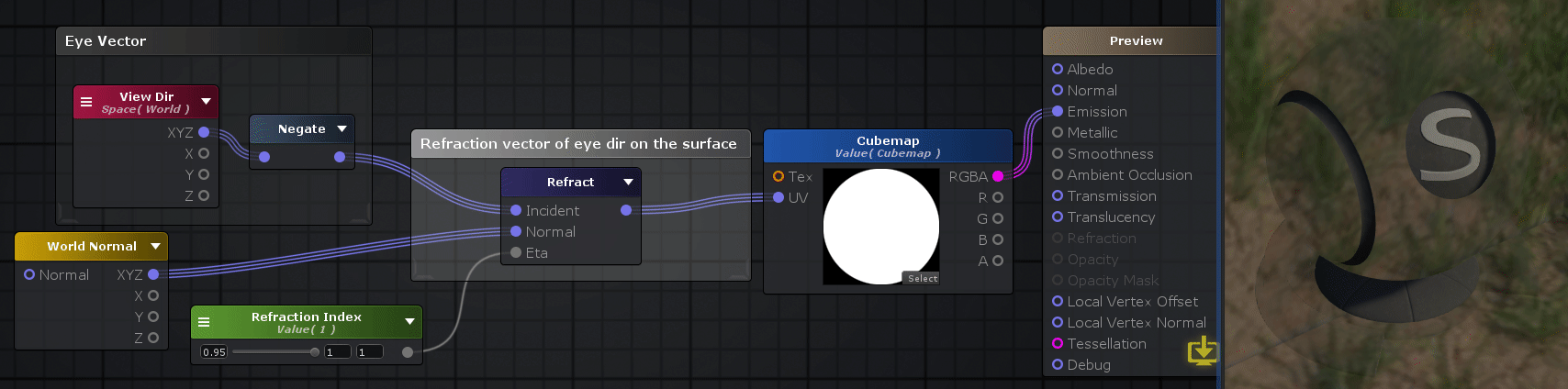## Refract Node

The Refract node calculates a refraction vector from a given Incident vector, a surface Normal vector and a ratio of Index of Refractions at the surface's interface. Both Normal and Incident vector should be normalized.

A cast is done to match the input type with the most amount of channels if Normal and Incident vectors have different channel amounts. This will also determine the refracted vector size.Nodes used: View Dir, Negate, World_Normal, Float, Refract, Texture Sample

Node Parameter Description Default Value
Incident Incident vector ( should be normalized beforehand ). Only visible if the respective input port is not connected. 0,0,0,0
Normal Normal vector ( should be normalized beforehand ). Only visible if the respective input port is not connected. 0,0,0,0
Eta Ratio of index of refraction, determines amount of incident ray that is refracted. Only visible if the respective input port is not connected. 0

Input Port Description Type
Incident Incident vector ( should be normalized beforehand ). Float4 
Normal Normal vector ( should be normalized beforehand ). Float4 
Eta Ratio of index of refraction, determines amount of incident ray that is refracted. Float

1. ^ Port automatically adapts to all connection types except Matrices and Sampler types.